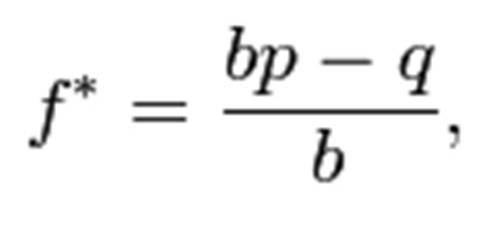In probability theory, the Kelly Criterion is a formula used to determine the optimal size of a series of bets. In most gambling scenarios, and some investing scenarios under some simplifying assumptions, the Kelly strategy will do better than any essentially different strategy in the long run. It was described by J. L. Kelly, Jr, in a 1956 issue of the Bell System Technical Journal.   Edward O. Thorp demonstrated the practical use of the formula in a 1961 address to the American Mathematical Society and later in his books Beat the Dealer (beating blackjack via card counting) and Beat the Market (with Sheen Kassouf, for investing). For simple bets with two outcomes, one involving losing the entire amount bet, and the other involving winning the bet amount multiplied by the payoff odds, the Kelly bet is:

where:

f* is the fraction of the current bankroll to wager
b is the net odds received on the wager
p is the probability of winning
q is the probability of losing, which is 1 − pHomeEdward O. ThorpArticles & ResearchMediaKelly Criterion